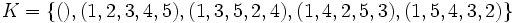# Sylow not implies NE

This article gives the statement and possibly, proof, of a non-implication relation between two subgroup properties. That is, it states that every subgroup satisfying the first subgroup property (i.e., Sylow subgroup) need not satisfy the second subgroup property (i.e., NE-subgroup)
View a complete list of subgroup property non-implications | View a complete list of subgroup property implications
EXPLORE EXAMPLES YOURSELF: View examples of subgroups satisfying property Sylow subgroup but not NE-subgroup|View examples of subgroups satisfying property Sylow subgroup and NE-subgroup

## Statement

A Sylow subgroup of a finite group need not be a NE-subgroup: it need not equal the intersection of its normalizer and normal closure in the whole group.

## Facts used

1. A5 is simple

## Proof

### Example of the alternating group of degree five

Further information: alternating group:A5

Let$G$ be the alternating group on$\{ 1,2,3,4,5 \}$. Then:

• Let$H$ be a$2$-Sylow subgroup of$G$, for instance,$H = \{ (), (1,2)(3,4), (1,3)(2,4), (1,4)(2,3) \}$. Since$G$ is simple, the normal closure of$H$ in$G$ is$G$. The normalizer of$H$ in$G$ is the alternating group on$\{ 1,2,3, 4\}$, which is strictly bigger than$H$. Thus, the intersection of the normalizer and normal closure is strictly bigger than$H$.
• Let$K$ be a$5$-Sylow subgroup of$G$, for instance,$K = \{ (), (1,2,3,4,5), (1,3,5,2,4), (1,4,2,5,3), (1,5,4,3,2) \}$. Then, the normalizer of$K$ in$G$ is a dihedral group of order ten, which in particular includes double transpositions such as$(2,5)(3,4)$. The normal closure of$K$ in$G$ is$G$. Thus, the intersection of the normalizer and normal closure is strictly bigger than$K$.